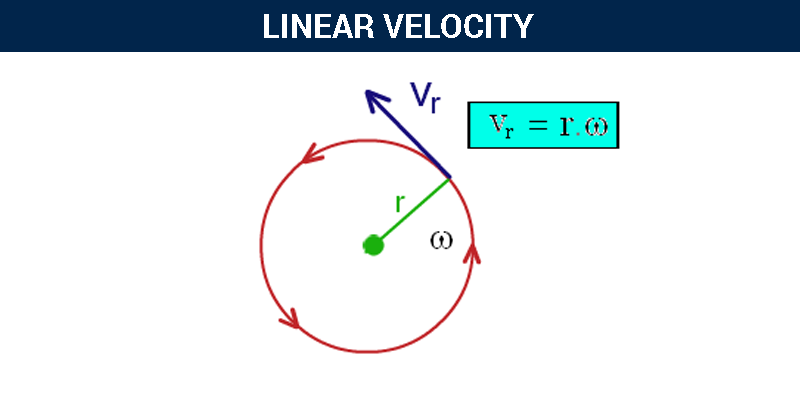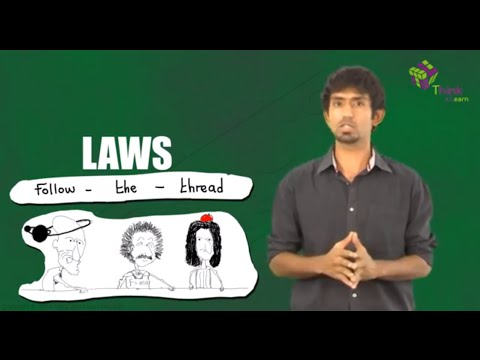# Linear Velocity

Velocity is the rate of change in the position of an object in a specific range of time. When the object moves along the straight path, the velocity associated with it is termed as linear velocity. It is given as the ratio of distance covered to time. Denoted using V or Vl and measure using SI unit m/s. It is a vector quantity.

## What is Linear Velocity?

Linear velocity is the measure of “the rate of change of displacement with respect to time when the object moves along a straight path.” It is a vector quantity. Dimension formula of linear velocity is [M]0[L]1[T]-1

Linear and angular velocities are related to the speed of an object based on the perspective chosen. Linear velocity is applied to an object that moves, whereas angular velocity applies to those that turn such as a wheel, the earth’s revolution or a spinning top.

### Linear Velocity Formula

The linear velocity depends on the distance travelled by an object with respect to time taken. The linear equation or the linear velocity formula is given by,

v = x/t

Where,

• v = Linear velocity
• x = distance covered
• t = Time taken to cover the distance(x).

### Linear Velocity units

Linear velocity is measured using SI unit meter per second or m/s

## Angular Velocity to Linear Velocity

Anything that moves or turns in the circular direction has both linear velocity and angular velocity. The angular velocity is the ratio of the angle traversed to the amount of time it takes to traverse that angle.Angular velocity gives an expression of angular displacement over time and can be expressed in radians or degrees. Angular velocity is determined with the above-mentioned equation.

To calculate the linear velocity from angular velocity, you can apply the formula, where –

r indicates the radius of the path taken.

### Constant Linear Velocity

When a body moves with constant speed without changing its direction, it is said to be moving with the constant linear velocity. Linear velocity indicates the direction is not changed, and constant shows that the magnitude remained constant.

## Linear Velocity Important Questions

Q1. What is linear velocity?

Ans: Linear velocity is defined as the vector quantity that denoted the rate of change of position of an object from its equilibrium position with respect to mass.

Q2. How to determine the magnitude of the linear velocity of a point B on a link AB which is relative to point A?

Ans: Following is the equation that is used to determine the magnitude of the linear velocity of a point B on a link Ab relative to point A:

ω*AB

Q3. What is a tangential component?

Ans: A tangential component is a component at which the centripetal component is parallel to the velocity of the particle.

Q4. What is a radial component?

Ans: A radial component is a component at which the centripetal component is perpendicular to the velocity of the particle.

Q5. What is the dimensional formula of angular momentum?

Ans: [ML2T-1]

Q6. What does the rate of displacement mean?

Ans: The rate of displacement means the velocity of the body which measured in metres per second.

Q7. What is instantaneous velocity?

Ans: Instantaneous velocity is the velocity of the body at a given instant. The SI unit of instantaneous velocity is m.s-1.

Q8. How to determine the direction of the linear velocity of a body moving in a circle?

Ans: The direction of a linear velocity of a body moving in a circle is determined along the tangent.

Q9. What is the angle between the linear velocity and the angular velocity of a body moving in a circle?

Ans: The angle between the linear velocity and the angular velocity of a body moving in a circle is 90°.

## More questions on Linear Velocity

Q1: Define linear velocity.

Ans:The rate of change of displacement with respect to time when the object moves along a straight path.

Q2: Write the expression for linear velocity.

Ans: the expression for linear velocity is $v=\frac{x}{t}$

Q3: Write the dimensional formula of linear velocity.

Ans: The dimensional formula of linear velocity is [M][L]1[T]-1

Q4: State true or False. “Linear velocity is a scalar quantity.”

Ans: False. Linear velocity is a vector quantity.

Q5: Write the type of motion involved in linear velocity.

Ans: Motion along a straight line

Q6: Write the SI unit of linear velocity.

Ans: SI Unit of linear velocity is m/s.

Q7: Name the types of velocities involved in a circular motion.

Ans: Angular velocity and linear velocity.

Q8: if a object covers 3 meters in 2 seconds along a straight. Calculate its linear velocity.

Ans: substituting the values in v = x/t , we get linear velocity as 1.5m/s.

Q9: A plane is travelling Northeast. If the eastern component of it’s velocity is 300 m/h, how fast is the plane travelling?

Ans: 424 m/h

Q10: An object is moving in a circular path. How will it’s linear velocity change if the diameter of the circular path was decreased by one half?

Ans: ½

Hope you have understood about linear velocity, How it is calculated, Its Formula along with terms, units.

Physics Related Topics:

Stay tuned with BYJU’S for more such interesting articles. Also, register to “BYJU’S-The Learning App” for loads of interactive, engaging physics related videos and an unlimited academic assist.